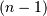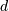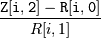# scipy.cluster.hierarchy.inconsistent¶

scipy.cluster.hierarchy.inconsistent(Z, d=2)[source]

Calculates inconsistency statistics on a linkage.

Note: This function behaves similarly to the MATLAB(TM) inconsistent function.

Parameters: Z : ndarray Theby 4 matrix encoding the linkage (hierarchical clustering). See linkage documentation for more information on its form. d : int, optional The number of links up to d levels below each non-singleton cluster. R : ndarray Aby 5 matrix where the i‘th row contains the link statistics for the non-singleton cluster i. The link statistics are computed over the link heights for linkslevels below the cluster i. R[i,0] and R[i,1] are the mean and standard deviation of the link heights, respectively; R[i,2] is the number of links included in the calculation; and R[i,3] is the inconsistency coefficient,